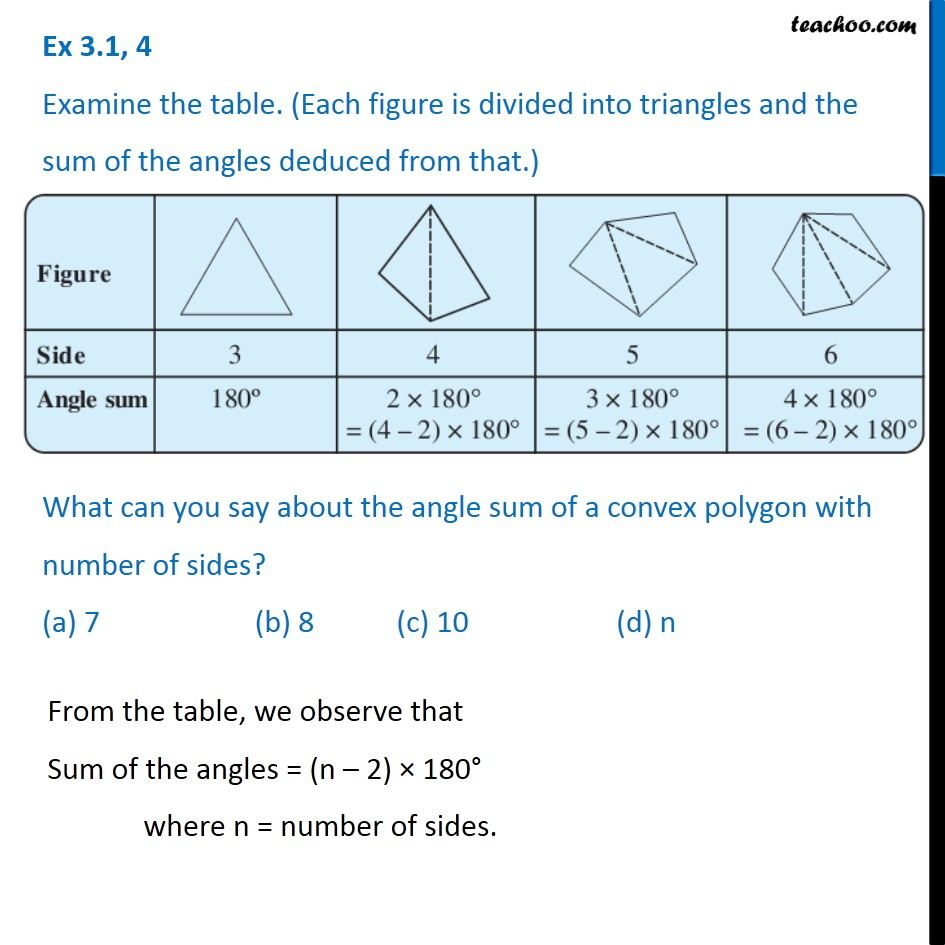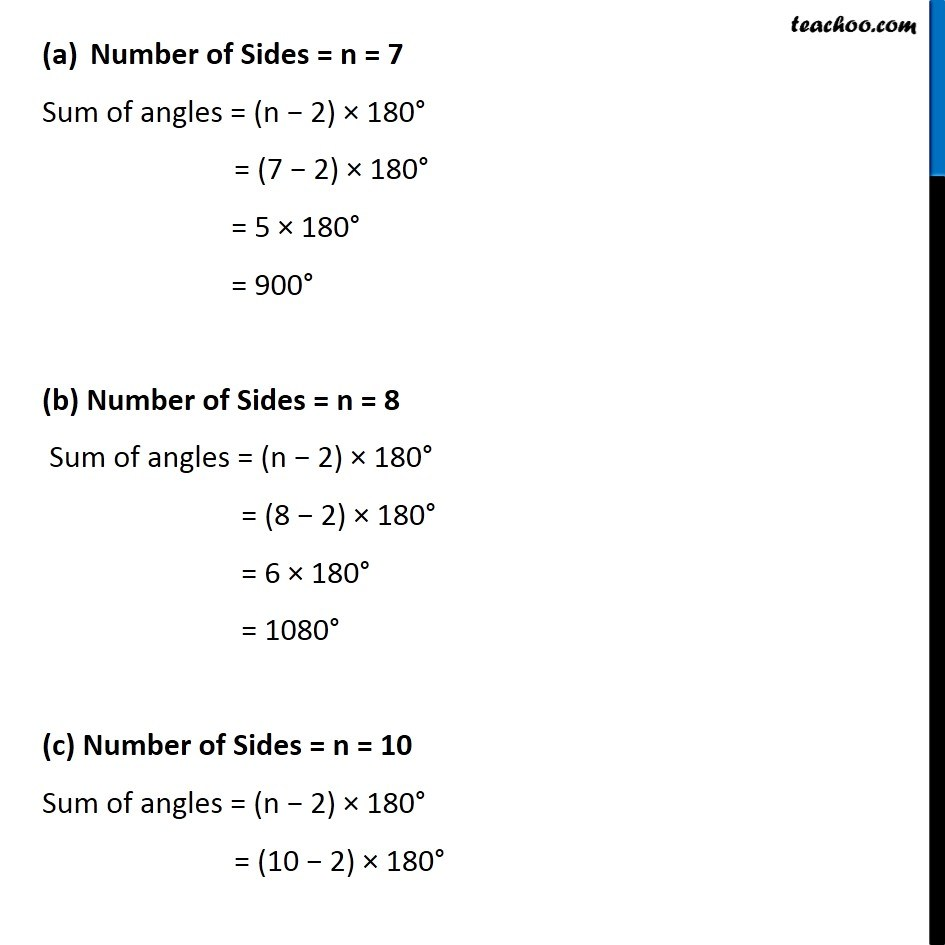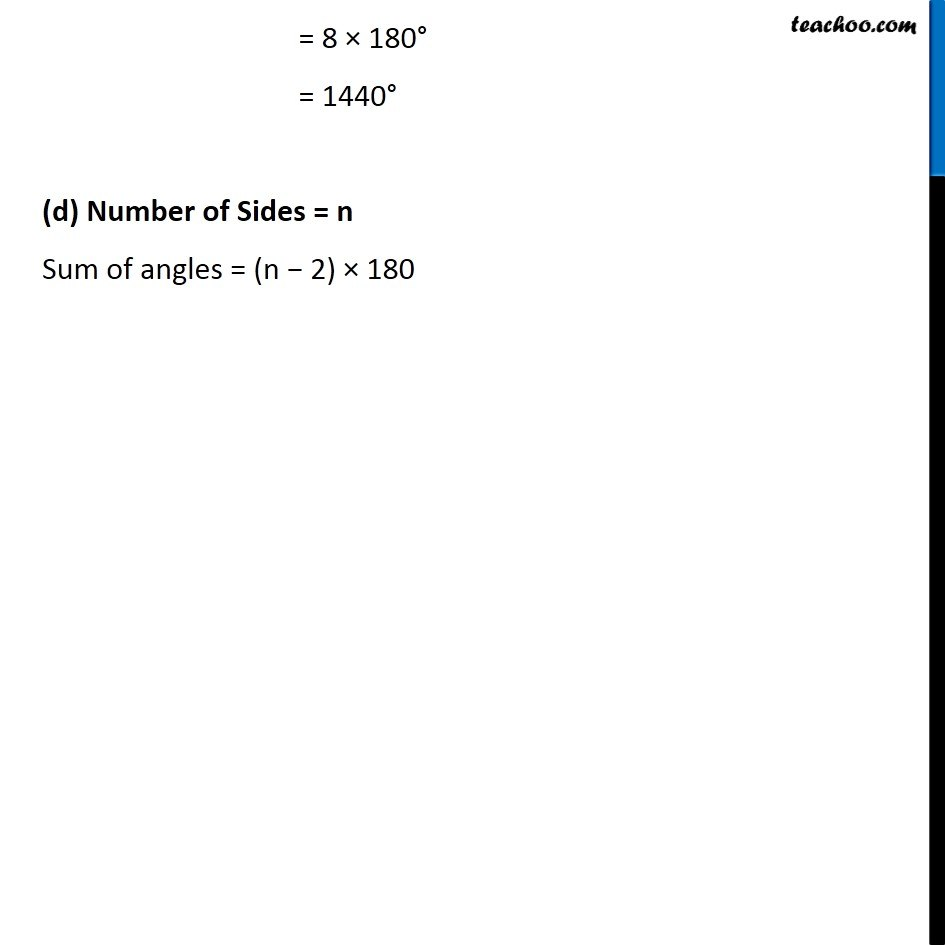Polygons

Chapter 3 Class 8 Understanding Quadrilaterals
Concept wiseLearn in your speed, with individual attention - Teachoo Maths 1-on-1 Class

### Transcript

Question 3 Examine the table. (Each figure is divided into triangles and the sum of the angles deduced from that.) What can you say about the angle sum of a convex polygon with number of sides? (a) 7 (b) 8 (c) 10 (d) n From the table, we observe that Sum of the angles = (n – 2) × 180° where n = number of sides. Number of Sides = n = 7 Sum of angles = (n − 2) × 180° = (7 − 2) × 180° = 5 × 180° = 900° (b) Number of Sides = n = 8 Sum of angles = (n − 2) × 180° = (8 − 2) × 180° = 6 × 180° = 1080° (c) Number of Sides = n = 10 Sum of angles = (n − 2) × 180° = (10 − 2) × 180° = 8 × 180° = 1440° (d) Number of Sides = n Sum of angles = (n − 2) × 180